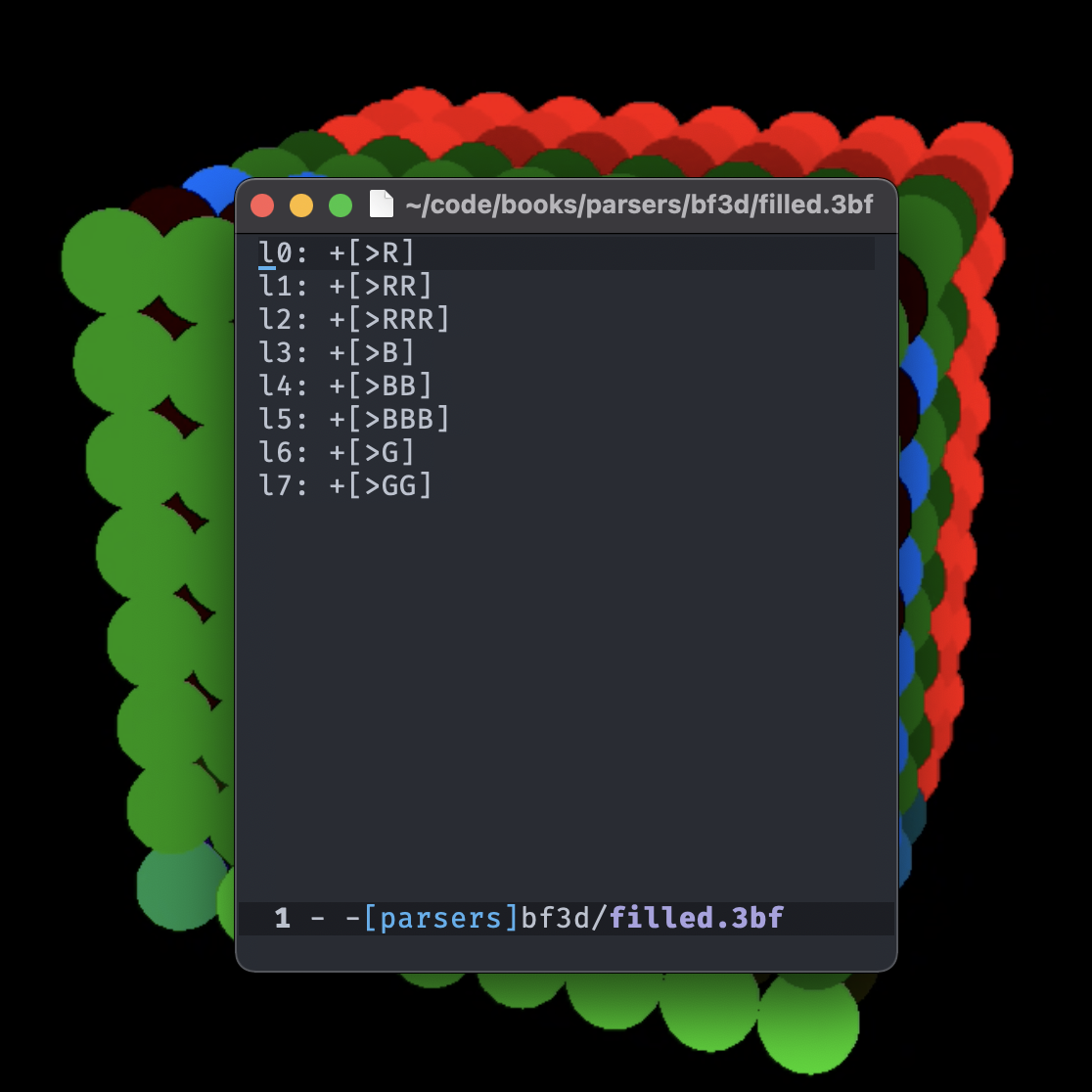# BF3D_Video depicting a Bf3d program running as it fills in a diamond shaped 3d shape on the screen_

Bf3d is a simple esoteric programming language inspired by BrainFuck and like languages. Bf3d uses the same basic syntax as BrainFuck, but replaces `+` and `-` with `R` `G` and `B` which increment colors and `r` `g` `b` to decrement a color. Also, because the program works in 3 dimensions, the program can move the cursor left and right with `>` and `<` like usual, but the cursor can also move up and down with `^` and `v`. Each cell is represented as an 8-bit number. An additional keyword `l` followed by a number specifying the layer that the program operates over.

``````RRRGGGBB
00000000
``````

8-bit color format

## Technical details

This compiler uses `flex` and `lemon` to lex and parse the program. The program is then stored in a bytecode format in memory that is then run following the rules of the program until it completes. The graphics are done using `raylib`.

## Example Program

To draw the diamond in the example above, one would write the program:

``````l0:>>>>^^^^+.
l1:+[>>>>^^^^[
>BB
<^BB
<VBB
V>BB
^.-<<<<VVVV-]<<<<VVVV]

l2:+[>>>>^^^^[
>>BBB
<^BBB<^BBB
<VBBB<VBBB
V>BBBV>BBB
>^BBB
^<.-<<<<VVVV-]<<<<VVVV]

l3:+[>>>>^^^^[>>>GGG
^<GGG^<GGG^<GGG
V<GGGV<GGGV<GGG
V>GGGV>GGGV>GGG
^>GGG^>GGG
<<^.-<<<<VVVV-]<<<<VVVV]

l4:+[>>>>^^^^[>>>GG
^<GG^<GG^<GG
V<GGV<GGV<GG
V>GGV>GGV>GG
^>GG^>GG
<<^.-<<<<VVVV-]<<<<VVVV]

l5:+[>>>>^^^^[
>>RR
<^RR<^RR
<VRR<VRR
V>RRV>RR
>^RR
^<.-<<<<VVVV-]<<<<VVVV]

l6:+[>>>>^^^^[
>RRR
<^RRR
<VRRR
V>RRR
^.-<<<<VVVV-]<<<<VVVV]
l7:+[>>>>^^^^[-RRRR <<<<VVVV-]<<<<VVVV]
``````